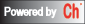## Time-Domain Response Analysis

The time-domain approach is a unified method for analyzing and designing systems modeled by either modern or classical approach. Time-domain response analysis functions below can be used to study the output behavior of a linear time-invariant system driven by system inputs and initial states.

The step or impulse response plots the system response with respect to time when the system input is a step or impulse function, respectively. The initial response plots the system response to the initial state x0 with zero-input. The simulation response plots the system response to any input and initial state specified by the user. The initial state of zero is assumed by default.

Function
`    `
Description
Step response Plot step response of a system in time domain.
Impulse response Plot impulse response of a system in time domain.
Initial response Plot time domain initial response of a system represented in state space.
Simulation response Simulate system response to an arbitrary input.